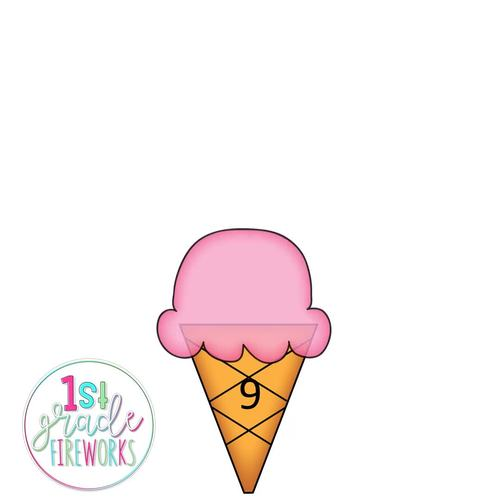Subject
Resource Type
File Type
PDF (1 MB|33 pages)
Standards
\$2.00
• Product Description
• Standards

Addition math fact cards for students to match & CREATE.

Students will sort addition math facts ( to 10) to their sum.

Facts 11-20 can be made by students. Card frames available.

Ice Cream themed. Available in color & B/W.

Apply properties of operations as strategies to add and subtract. If 8 + 3 = 11 is known, then 3 + 8 = 11 is also known. (Commutative property of addition.) To add 2 + 6 + 4, the second two numbers can be added to make a ten, so 2 + 6 + 4 = 2 + 10 = 12. (Associative property of addition.)
Total Pages
33 pages